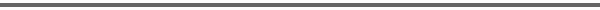Journal Home Page Complete Contentsof this Volume Previous Article Next Article Journal of Lie Theory 14 (2004), No. 2, 317--338 Copyright Heldermann Verlag 2004Tits Geometry, Arithmetic Groups, and the Proof of a Conjecture of Siegel Enrico Leuzinger Mathematisches Institut II, Universität Karlsruhe, Englerstr. 2, 76131 Karlsruhe, Germany, enrico.leuzinger@math.uni-karlsruhe.de[Abstract-pdf] Let $X = G/K$ be a Riemannian symmetric space of noncompact type and of rank $\geq 2$. An irreducible, non-uniform lattice $\Gamma\subset G$ in the isometry group of $X$ is arithmetic and gives rise to a locally symmetric space $V=\Gamma\backslash X$. Let $\pi:X\rightarrow V$ be the canonical projection. Reduction theory for arithmetic groups provides a dissection $V=\coprod_{i=1}^k \pi(X_i)$ with $\pi(X_0)$ compact and such that the restiction of $\pi$ to $X_i$ is injective for each $i$. In this paper we complete reduction theory by focusing on metric properties of the sets $X_i$. We detect subsets $C_i$ of $X_i$ (${\Bbb Q}$--Weyl chambers) such that $\pi_{\mid C_i}$ is an isometry and such that $C_i$ is a net in $X_i$. This result is then used to prove a conjecture of C.L. Siegel. We also show that $V$ is quasi-isometric to the Euclidean cone over a finite simplicial complex and study the Tits geometry of $V$. [FullText-pdf (241 KB)] for subscribers only.